# Kindergarten Math Worksheets

### Challenge your kindergarteners to math worksheets that will inspire them to learn to be creative as they count by 5s, 10s, learn numbers to 100, and start to add and subtract small numbers. Lots of handwriting number practice and fun math problems to solve in these kindergarten math worksheets.Free Kindergarten Math Worksheets:Math Worksheet Practice Weekly Workbook FreebieKindergarten Math and Critical Thinking WorksheetsEasy Kindergarten Math Worksheets Workbook

Kindergarten Geometry

Order Numbers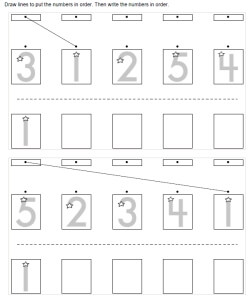Ordering five numbers (numbers 1 to 5) Ordering five numbers (numbers can be from 1 to 9)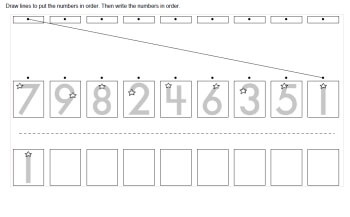Ordering nine numbers (numbers 1 to 9)

Counting

Number Lines

Calendar Skills:  Practice Writing a Calendar Each Month

Comparing Numbers

Addition and Introduction to Addition

Subtraction and Introduction to Subtraction

Color by Number

Color by Addition

Color by Subtraction

Skip Counting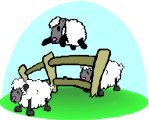Skip Counting Reading Comprehensions (grades 1-2 reading level)

Introduction to Math
 Mazes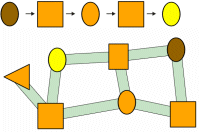Number Mazes and Shapes Mazes

 Matching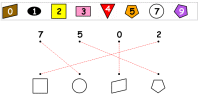Matching Shapes, Numbers, and Colors

 Combined Shapes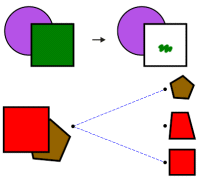Combined Shapes: Coloring and Matching

 Ordering Animals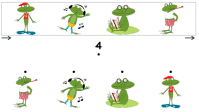Ordering Animals

 Ordering Shapes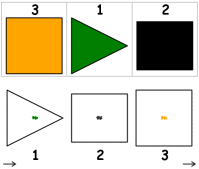Ordering Shapes

 Counting - Part 1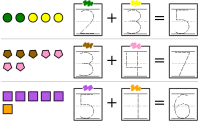Math Counting (part 1)

 Counting - Part 2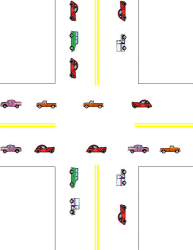Math Counting (part 2)

 Grids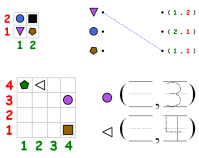Grids: Shapes, Positions, Writing Numbers

 Greater and Less Than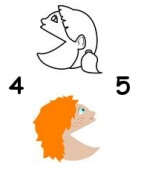Greater and Less Than

Hundreds Chart

Hundreds Chart Pieces Puzzle

Have a suggestion or would like to leave feedback?
Leave your suggestions or comments about edHelper!